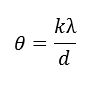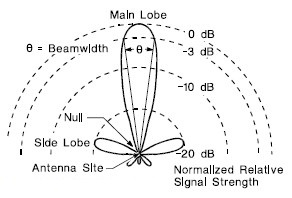# Antenna Beamwidth Calculator

This CalcTown calculator calculates the half power beam width (HPBW) of a parabolic antenna.

The angular width of the beam radiated by high-gain antennas is measured by the half-power beam width (HPBW), which is the angular separation between the points on the antenna radiation pattern at which the power drops to one-half (-3 dB) its maximum value. For parabolic antennas, the HPBW, ? is given by:

m

#### Result

radiansClick here to view image

Where,

λ = Wavelength

d = Diameter of the antenna aperture

k = Factor which varies slightly depending on the shape of the reflector and the feed illumination pattern.

θ = Parabolic antenna beam-width

'k' is a factor which varies slightly depending on the shape of the reflector and the feed illumination pattern. For an ideal uniformly illuminated parabolic reflector and θ in degrees, k would be 57.3 (the number of degrees in a radian). For a "typical" parabolic antenna k is approximately 70.